Home

# Rational points on Erdős–Selfridge superelliptic curves

## Abstract

Given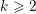$k\geqslant 2$ , we show that there are at most finitely many rational numbers$x$ and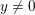$y\neq 0$ and integers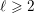$\ell \geqslant 2$ (with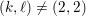$(k,\ell )\neq (2,2)$ ) for which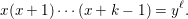$$\begin{eqnarray}x(x+1)\cdots (x+k-1)=y^{\ell }.\end{eqnarray}$$
In particular, if we assume that$\ell$ is prime, then all such triples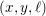$(x,y,\ell )$ satisfy either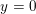$y=0$ or$\ell <\exp (3^{k})$ .

## References

Hide All
MathJax
MathJax is a JavaScript display engine for mathematics. For more information see http://www.mathjax.org.

# Rational points on Erdős–Selfridge superelliptic curves

## Metrics

### Full text viewsFull text views reflects the number of PDF downloads, PDFs sent to Google Drive, Dropbox and Kindle and HTML full text views.

Total number of HTML views: 0
Total number of PDF views: 0 *Loading metrics...

### Abstract viewsAbstract views reflect the number of visits to the article landing page.

Total abstract views: 0 *Loading metrics...

* Views captured on Cambridge Core between <date>. This data will be updated every 24 hours.

Usage data cannot currently be displayed